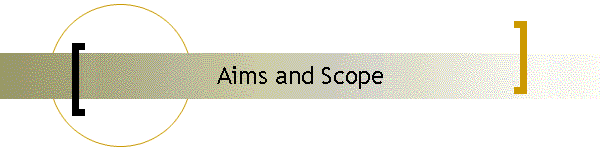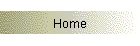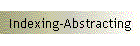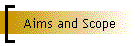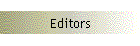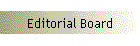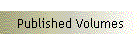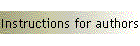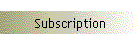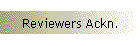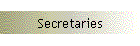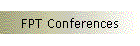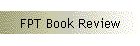Fixed Point Theory publishes relevant research and expository papers devoted to the all topics of fixed point theory  and applications in all structured set (algebraic, metric, topological (general and algebraic), geometric (synthetic, analytic, metric, differential, topological), ...) and in category theory. Applications to ordinary differential equations, partial differential equations, functional equations, integral equations, mathematical physics, mathematical chemistry, mathematical biology, mathematical economics, mathematical finances, informatics, ..., are  also welcome. The journal FIXED POINT THEORY adheres to the EMS Code of Practice and endorses the Best Current Practices for Journals approved by IMU.

Home | Indexing-Abstracting | Aims and Scope | Editors | Editorial Board | Published Volumes | Instructions for authors | Subscription | Reviewers Ackn. | Secretaries | FPT Conferences | FPT Book Review